Students get through the TN Board 12th Economics Important Questions Chapter 4 Consumption and Investment Functions which is useful for their exam preparation.

## TN State Board 12th Economics Important Questions Chapter 4 Consumption and Investment Functions

Question 1.
What do you mean by investment function?
It is investment – interest rate relationship. I = f(R) I – Investment (Dependent variable) R – Rate of interest (Independent variable).

Question 2.
What do you mean by windfall gains or losses?
Unexpected changes in the stock market leading to gains or losses tend to shift the consumption function upward or downward.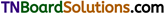Question 3.
What do you mean by investment?
Investments mean the purchase of stocks and shares, debentures, government bonds, and equities.

Question 4.
What is investment according to Keynes?
According to Keynes, it is an only a financial investment and not a real investment. This type of investment does result in an addition to tHe stock of real capital of the Nation.

Question 5.
What is Autonomous investment?
It is the expenditure on capital formation, which is independent of the change in income, rate of interest or rate of profit.

Question 6.
Write a note on autonomous investment.
Autonomous investment is one of the key concepts in welfare economics. In times of economic depression, the governments try to boost autonomous investments.

Question 7.
What do you mean by induced investment?
Induced investment is the expenditure on fixed assets and stocks which are required when the level of income and demand in any economy goes up.Question 8.
MEC depends on two factors. What are they?
The prospective yield from a capital asset and the supply price of a capital asset.

Question 9.
Name the factors that are taken into consideration while making any investment decisions.
MEC:

1. The cost of the capital asset
2. The expected rate of return from MEC during its lifetime
3. The market rate of interest

Question 10.
What is full employment situations?
Under the conditions of full employment, resources are fully employed. So additional investment will lead to inflation only, rather than the generation of additional income.

Question 11.
Name the kinds of multipliers.
Tax multipliers, employment multipliers, Foreign trade multipliers, and Investment multipliers.

Question 12.
What are the accelerator effects?

1. Increase in consumer demand.
2. Films get close to full capacity.
3. Films invert to meet rising demand.

Question 13.
What are Super multipliers?
The Super multipliers are greater than the simple multiplier which includes only autonomous investments and no induced investment, while super multipliers include investments.Question 1.
What are the features of autonomous investments?

1. Investments that are not dependent on National income.
2. Mainly done with the welfare motive and not for making profits.
3. Example: Construction of roads and bridges.
4. Not affected by rest in raw material or wages of workers.
5. Essential to the development of Nation and out of depression.

Question 2.
Write a note on Induced investments.
It is profit-motivated. It is related to the changes in national income. The relationship between the National income and Induced investment is positive, decreases in National income lead to a decrease in induced investment and vice versa. Induced investment is income elastic. It is positively sloped.

Question 3.
Write a note on the marginal efficiency of capital.
It is the rate of discount which makes the discount present value of the expected income stream equal to the cost of capital. It was first introduced by J.M.Keynes in 1936 as an important determinant of Autonomous investment. The MEC is the expected profitability of an additional capital asset.Question 4.
Differentiate – Positive and Negative multiplier.

 Positive multiplier Negative multiplier When an initial increases in injection (or a decrease in a leakage) leads to a greater final increase in real GDP. When an initial increases in an injection ( or an increase in a leakage) leads to a greater final decrease in real GDP.

Question 5.
Differentiate between the static multiplier and dynamic multiplier.

 Static multiplier Dynamic multiplier It is known as simultaneous multiplier timeless and logical multiplier. The change in investment and the resulting change in income are simultaneous. It is also known as sequence multiplier. There is also change in MPC as the economy moves from one equilibrium position to another one. In real life income level doesn’t increase instantly with investment. In fact, there is a time lag between increase in income and consumption expenditure.

Question 6.
What are the assumptions of the accelerator?

1. Absence of excess capacity in consumer goods industries.
2. Constant capital-output ratio.
3. An increase in demand is assumed to be permanent.
4. The supply of funds and other inputs is quite elastic.
5. Capital goods are perfectly divisible in any required size.Question 1.
Explain consumption function.
It is the functional relationship between two aggregates i.e., Total consumption and Gross national income. C = f(Y).
C = Consumption, Y = Income, f = Function. It is the relationship between C and Y C = dependent Y = independent variables. It is also known as MPC. The consumption function is a schedule of the various amounts of consumption expenditure corresponding to different levels of income.

 Income Y Consumption C Savings S 0 20 -20 60 70 -10 120 120 0 180 170 10 240 220 20 300 270 30 360 320 40

If we take C = 100 + 0.8Y then MPC = 0.8. Here if Y = 0, C = 100, If
Y = 100, C = 180. If Y = 200, C = 260. If Y = 300, C = 340 (MPC = $$\frac{\Delta \mathrm{C}}{\Delta \mathrm{Y}}$$ = 0.8). In mathematical terms, C = a + by or C = 20 + 0.8Y where a > 0 and b < 1.
C = Consumption
A = Constant or intercept
Y = Income
b = MPC = $$\frac{\Delta \mathrm{C}}{\Delta \mathrm{Y}}$$
Here, when Y = 120, C = 120 (Point B is the diagram)
When Y = 180, C = 170, S = 10 (Point S is the diagram)
If Y increases to 360, C = 320, S = 40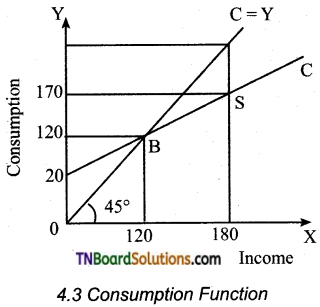In the diagram, income is measured on X-axis and consumption on Y-axis. In the 45° line at all levels, income and consumption are equal. It is a linear consumption. The 45° line is regarded as a zero-saving line. It measures both amounts consumed and saved. The shape of the line indicates the division of income between consumption and saving.Question 2.
Write the determinants of the investment function.
According to classical economists, investments depend exclusively on the rate of interest. But in reality, investments depend on a No. of factors. They are

1. Rate of interest
2. Level of uncertainty
3. Political environment
4. Rate of growth of population
5. The stock of capital goods
6. The necessity of new products
7. Level of income of investors
8. Inventions and innovations
9. Consumer demand
10. Policy of the state
11. Availability of capital
12. Liquid assets of the investors

Keynes contended that business expectations and profits are more important in deciding investment. He also pointed out that investment depends on MEC and the rate of interest.

Question 3.
Explain the factors on which MEC is dependent.
Marginal Efficiency of capital depends on two factors
(i) The prospective yields from a capital asset
(ii) The supply price of a capital asset The MEC is influenced by
(a) Short-run factors
(b) long-run factors
(a) Short-run factors:

1. Demand for products: The rate of return from the investment will be high if the market for a particular good grows and costs are likely to fall. But if entrepreneurs expect a fall in demand for goods and a rise in cost, the investment will decline.
2. Liquid assets: If entrepreneurs have more capital, thus take advantage of more investment.
3. Sudden change in income: If the income of the entrepreneur increases, there will be a high investment in the country.
4. The current rate of investment: If the rate of investment in a particular industry is very large, the MEC will below.
5. Waves of optimism and pessimism: When businessmen are optimistic, MEC increases and when they are pessimistic, MEC is low.(b) Long-run factors:

1. Rate of growth of population: When the population grows rapidly, MEC will increase and investment is discouraged and MEC will reduce.
2. Technological progress: This will increase investment and MEC.
3. Monetary and fiscal policies: The policy of cheap money and liberal tax will give more profit and MEC will be high.
4. Political environment: The political stability and administration will improve MEC.
5. Resource availability: The supply of more Natural resources will increase the Marginal Efficiency of Capital.

Question 4.
Write the assumption of the multiplier.
Assumptions of Keynes theory of multiplier.

1. There is a change in autonomous investment.
2. The marginal propensity to consume is constant.
3. There is no induced investment.
4. Consumption is a function of current income.
5. Consumer goods are available in response to effective demand for them.
6. There is a closed economy unaffected by foreign influences.
7. There are no changes in prices.
8. There is less than full employment level in the economy.

Question 5.
Explain the relationship between the rate of interest and investment.
Higher rates of interest will reduce the investment because higher rates increase the cost of borrowing and require investment to have a higher rate of return to be profitable.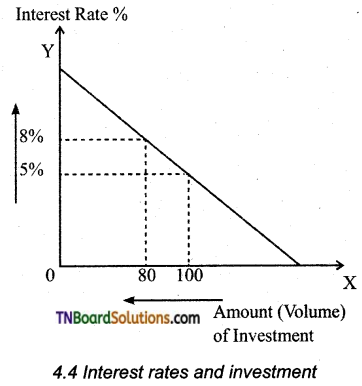Explanation of the diagram:
When the real cost of borrowings rises, few investment projects are profitable. If interest rates increase from 5% to 8%, then we get a fall in the amount of investment from Rs. 100cr to Rs. 80cr. The increase in interest rates will discourage investment because investment has a higher opportunity cost.

1. It is more expensive to borrow money from the bank when interest rates are higher.
2. Saving money in the bank gives a higher rate of return. So using savings to finance investment has an opportunity cost of lower interest payments.
3. If interest rates increase, firms will gain a better rate of return to justify the cost of borrowing using savings.Question 6.
Explain MEC and Multiplier.
The MPC refers to the relation between change in consumption (c) and change in income(Y).
Symbolically MPC = $$\frac{\Delta \mathrm{C}}{\Delta \mathrm{Y}}$$
The value of the multiplier depends on MPC.
Multiplier (K) = 1/1-MPC
The multiplier is the reciprocal of one minus marginal propensity to consume. Since marginal propensity to save is 1 – MPC. (MPC+MPS =1). The multiplier is 1/ MPS. The multiplier is therefore defined as reciprocal of MPS. The multiplier is inversely related to MPS and directly with MPC.
Numerically if MPC is 0.75, MPS is 0.25 and k is 4.
Using formula K = 1 /1 – MPC
1 / 1 – 0.75 = 1 / 0.25 = 4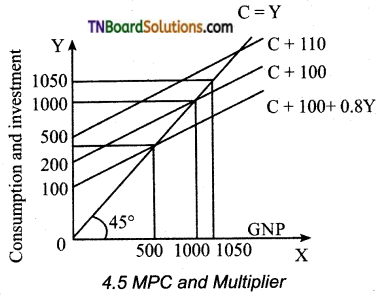Taking the following values, we can explain the functioning of multiplier.

 MPC MPS K 0.00 1.00 1 0.10 0.90 1.11 0.50 0.50 2.00 0.75 0.25 4.00 0.90 0.10 10.00 1.00 0.00 α

C = 100 + 0.8 Y; I = 100
I = 10
Y = C + I
= 100 + 0.8Y + 100
0.2Y = 200
Y = 1000
Here, C = 100 + 0.8Y = 100 + (1000) = 900;
S = 100 = I
After I is raised by 10, now I = 110,
Y = 100 + 0.8Y + 110 .
0.2Y = 210
Y = $$\frac{210}{0.2}$$ = 1050
Here C = 100 = 0.8 (1050) = 940; S = 110 = I

Diagrammatic Explanation:
At 45° line Y = C + S
It implies the variables in axis and axis are equal.
The MPC is assumed to be at 0.8
(C = 100 + 0.8Y)
The aggregate demand (C + I) curve intersects 45° line at point E.
The original national income is 500.
(C = 100 + 0.8Y = 100 + 0.8 (500) = 500)
When I is 100, Y = 1000, C = 900;
S = 100 = I
The new aggregate demand curve is
C+I =100 +0.8y+100+10
Y = $$\frac{210}{0.2}$$ = 1050
C = 940; S = 110 = IQuestion 7.
Explain the leakage of the multiplier.
The multiplier assumes that those who earn income are likely to spend a proportion of their additional income on consumption. But in practice, people spend it on other items such expenses are known as leakages.

1. Payment towards past debts: Repayment of old loans, then MPC is reduced and the value of the multiplier is cut.
2. Purchase of existing wealth: Income is used for the purchase of land, buildings, etc., the money is circulated among people and does not consume. So the value of the multiplier is affected.
3. Import of goods and services: This money spent goes out of the country. Thus, imports reduce the value of the multiplier.
4. Nonavailability of consumer goods: According to multiplier theory supply of consumer goods following demand. But there is often a time lag. During this gap (d > s). inflation increases. So the consumption expenditure and the multiplier value decreases.

Question 8.
Write the uses of multipliers.

1. Multiplier highlights the importance of investment in income and employment theory.
2. It gives importance to the different stages of the trade cycle.
3. It also helps in bringing equality between S and I.
4. It helps in formulating government policies.
5. Multipliers help to reduce unemployment and achieve full employment.

Activity
Question 1.
How do you calculate MPC from the consumption function?
Consumption function or Propensity to consume. It is between two aggregates that is
(i) Total consumption and
(ii) Gross national income
C = f( Y)
MPC is the ratio of change iaconsumption to a change in income MPC = ΔC/ΔY
Consumption function is C = C0 + bY
(i) C0 is called autonomous consumption that is consumption is positive at zero levels of income.
(ii) The coefficient b measures the slope of consumption (The slope of consumption function gives the increase in consumption per unit increase in income). This is Marginal Propensity to Consume. So whenever there is an increase in consumption function, the MPC changes or MPC increases. Consumption changes by b for every rupee one change in income.
Solution:
Consumption changes in the same direction as income C = a + bY
C Consumption, a = Autonomous consumption (when the income is zero). b = slope of the consumption curve and Y = income. MPC is the ratio change in consumption to change in income. MPC = ΔC / ΔY
The value of MPC always lies between 0 and 1 that is 0 < MPC < 1. When income increases, then MPC also increases.
Propensity to consume: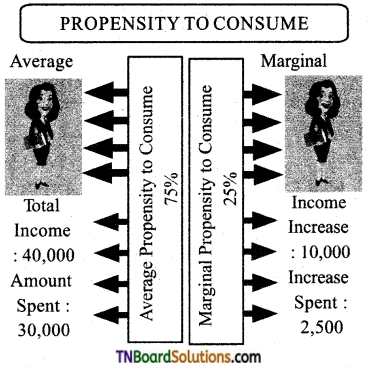Multiple choice questions

1. Consumption function and investment function play a vital role in influencing:
(a) MPC
(b) MPS
(c) NFIA
(d) National income
(d) National income

2. The consumption function is the relationship between the consumption expenditure and the:
(a) Per capita Income
(b) National income
(c) NFIA
d) Disposable income
(b) National income

3. In consumption function, the 45° line is regarded as:
(a) Price line
(b) Demand line
(c) Supply line
(d) Zero saving line
(d) Zero saving line

4. The keynes psychological law operates in ……….. economy
(a) Socialist
(b) Mixed
(d) Capitalist
(d) Capitalist5. According to keynes proposition of psychological law of consumption, when income increases, ………… also increases by smaller amount.
(a) Consumption expenditure
(b) Investment expenditure
(c) Savings
(d) Demand
(a) Consumption expenditure

6. J.M.Keynes has divided factors influencing the consumption function into:
(a) 4
(b) 2
(c) 3
(d) 5
(b) 2

7. Subjective factors are internal and related to ……….. feelings.
(a) Physical
(c) Negative
(d) Psychological
(d) Psychological

8. Objective factors are the factors.
(a) Internal
(b) Subjective
(c) External
(d) Normal
(c) External

9. ………. has made two observations regarding the factors affecting consumption.
(b) J.M.Keynes
(c) Duesenberry
(d) Alfred Marshall
(c) Duesenberry

10. There is a function and ……….. relationship between rate of interest and investment.
(a) Positive
(b) Inverse
(c) Normal
(d) Straight
(b) Inverse11. The autonomous investment curve is:
(a) Upward sloping
(b) Downward sloping
(c) Horizontal
(d) Vertical
(c) Horizontal

12. Generally government makes autonomous investment because of ………… consideration.
(a) Human
(b) Welfare
(c) Ospecious
(d) Individual
(b) Welfare

13. induced investment is income elastic and it is ………… sloped.
(a) Negatively
(b) Upward
(c) Downward
(d) Positively
(d) Positively

14. Induced investment is ………. motivated.
(a) Profit
(b) Sales
(c) Loss
(d) Production
(a) Profit

15. The classical economist believed that investment depends on:
(a) Rate of savings
(b) Rate of investment
(c) Rate of interest
(d) Rate of consumption
(c) Rate of interest16. MEC was 1st introduced by J.M.keynes in:
(a) 1936
(b) 1966
(c) 1956
(d) 1996
(a) 1936

17. MEC is also influenced by the rate of growth of:
(a) Agriculture
(b) Industries
(c) Population
(d) Supply
(c) Population

18. The concept of multiplier was first developed by:
(a) J.M.Keynes
(b) R.F.Khan
(c) Ricardo
(d) Samuelson
(b) R.F.Khan

19. Under static multiplier, the change in investment and the resulting change in income are:
(a) Continuous
(b) Discontinuous
(c) Simultaneous
(d) Changing
(c) Simultaneous20. Dynamic multiplier is also known as:
(a) Super multiplier
(b) Sequence multiplier
(c) Timeless multiplier
(d) Logical multiplier
(b) Sequence multiplier

21. The additional income spent on other items are known as:
(a) Wealth
(b) Inflation
(c) Investment
(d) Leakages
(d) Leakages

22. …….. reduces the value of the multiplier.
(a) Demand
(b) Imports
(c) Exports
(d) Supply
(b) Imports

23. Simple accelerator model was made by J.M.CIark in:
(a) 1915
(b) 1916
(c) 1917
(d) 1918
(c) 191724. The combined effect of the multiplier and the accelerator is also called as:
(a) Veblen effect
(c) Leverage effect
(d) Multiplier effect
(c) Leverage effect

25. Theory of income determination is based on:
(a) Ex-ante variable
(b) Ex-post variable
(c) Both (a) and (b)
(d) None of the above
(a) Ex-ante variable

26. MPC equals to:
(a) C/Y
(b) ΔC / ΔY
(c) 1 – C/Y
(d) ΔC / ΔY
(b) ΔC / ΔY

27. MPS equals to:
(a) 1 – MPC
(b) ΔS/ΔY
(c) Δ(Y – C) / ΔY
(d) All the above
(d) All the above

28. Aggregate consumption expenditures includes this type of expenditure:
(a) Autonomous
(b) Induced
(c) Accumulation
(d) Both autonomous and induced
(d) Both autonomous and induced29. Aggregate saving in the economy is:
(a) Positive throughout
(b) Positive and then negative
(c) Negative throughout
(d) Negative initially and then positive
(d) Negative initially and then positive

30. The Consumption function curve is:
(a) Upward sloping
(b) Downward sloping
(c) Parallel to the X-axis
(d) Parallel to the Y-axis
(a) Upward sloping

31. When AD exceeds AS, the inventories tend to:
(a) Rise
(b) Fall
(c) Rise initially and fall
(d) Remain unchanged
(b) Fall

32. Value of multiplier is determined by:
(a) MPS
(b) MPC
(c) Both (a) and (b)
(d) Investment
(c) Both (a) and (b)

33. If MPC = MPS, the value of multiplier is:
(a) 0
(b) 1
(c) 2
(d) Infinity
(c) 2Pick the odd one out.

1. …………… plays a vital role in influencing national income.
(a) Consumption function
(b) Investment function
(c) Consumption
(d) Price
(d) Price

2. ………… is a functional relationship between two aggregates.
(a) Consumption function
(b) Propensity function
(c) Aggregate demand
(d) Total consumption
(c) Aggregate demand

3. The Average Propensity to Consume =
(a) C/Y
(b) S/Y
(c) ΔC / ΔY
(d) dY / dX
(d) dY / dX4. The economy is faced with abnormal circumstances like:
(a) War
(b) Revolution
(c) Hyperinflation
(d) Habits
(d) Habits

5. The constant variables are:
(a) Tastes
(b) Social customs
(c) Income distribution
(d) Profit
(d) Profit

6. The unforeseen contingencies are:
(a) Accident
(b) Injury
(c) Sickness
(d) Demand
(d) Demand7. Keynes sums up the motives as:
(a) Precaution
(b) Calculation
(c) Foresight
(d) Income
(d) Income

8. Major objective factors influencing consumption functions are:
(a) Price level
(b) Wage level
(c) Supply level
(d) Interest rate
(c) Supply level

9. The term investment means:
(a) Purchase of stock and shares
(b) Debentures
(c) Government bonds
(d) Production
(d) Production

10. Private investment is an increase in the capital stock such as: